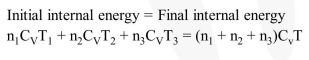Deepak Scored 45->99%ile with Bounce Back Crack Course. You can do it too!

# Three perfect gases at absolute

Question:

Three perfect gases at absolute temperatures $\mathrm{T}_{1}, \mathrm{~T}_{2}$ and $\mathrm{T}_{3}$ are mixed. The masses of molecules are $\mathrm{m}_{1}, \mathrm{~m}_{2}$, and $\mathrm{m}_{3}$ and the number of molecules are $\mathrm{n}_{1}, \mathrm{n}_{2}$ and $\mathrm{n}_{3}$ respectively. Assuming no loss of energy, then final temperature of the mixture is :-

1. $\frac{n_{1} T_{1}^{2}+n_{2} T_{2}^{2}+n_{3} T_{3}^{2}}{n_{1} T_{1}+n_{2} T_{2}+n_{3} T_{3}}$

2. $\frac{n_{1}^{2} T_{1}^{2}+n_{2}^{2} T_{2}^{2}+n_{3}^{2} T_{3}^{2}}{n_{1} T_{1}+n_{2} T_{2}+n_{3} T_{3}}$

3. $\frac{T_{1}+T_{2}+T_{3}}{3}$

4. $\frac{n_{1} T_{1}+n_{2} T_{2}+n_{3} T_{3}}{n_{1}+n_{2}+n_{3}}$

Correct Option: , 4

Solution: